# Coordinates

Determine the coordinates of the vertices and the content of the parallelogram, the two sides of which lie on the lines 8x + 3y + 1 = 0, 2x + y-1 = 0 and the diagonal on the line 3x + 2y + 3 = 0

x0 =  -2
y0 =  5
x1 =  1
y1 =  -3
x2 =  5
y2 =  -9
x3 =  8
y3 =  -17
S =  14

### Step-by-step explanation:

8x0+3y0+1=0
2x0+y0-1=0

8•x0+3•y0+1=0
2•x0+y0-1=0

8x0+3y0 = -1
2x0+y0 = 1

x0 = -2
y0 = 5

Our linear equations calculator calculates it.

8x1+3y1+1=0
3x1+ 2y1+3=0

8•x1+3•y1+1=0
3•x1+ 2•y1+3=0

8x1+3y1 = -1
3x1+2y1 = -3

x1 = 1
y1 = -3

Our linear equations calculator calculates it.

2x2+y2-1=0
3x2+ 2y2+3=0

2•x2+y2-1=0
3•x2+ 2•y2+3=0

2x2+y2 = 1
3x2+2y2 = -3

x2 = 5
y2 = -9

Our linear equations calculator calculates it.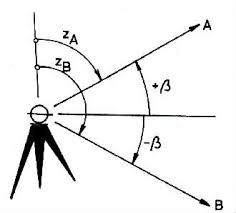Did you find an error or inaccuracy? Feel free to write us. Thank you!Tips to related online calculators
Line slope calculator is helpful for basic calculations in analytic geometry. The coordinates of two points in the plane calculate slope, normal and parametric line equation(s), slope, directional angle, direction vector, the length of the segment, intersections of the coordinate axes, etc.
Do you have a system of equations and looking for calculator system of linear equations?

#### You need to know the following knowledge to solve this word math problem:

We encourage you to watch this tutorial video on this math problem:

## Related math problems and questions:

• Triangle midpoints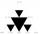Determine coordinates of triangle ABC vertices if we know tirangle sides midpoints SAB [0;3] SBC [1;6] SAC [4;5], its sides AB, BC, AC.
• Coordinates of the vertices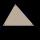Calculate the coordinates of the vertices of a triangle if the equations of its sides are 7x-4y-1 = 0 x-2y + 7 = 0 2x + y + 4 = 0
• Coordinates of the intersection of the diagonals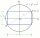In the rectangular coordinate system, a rectangle ABCD is drawn. The vertices of the rectangle are determined by these coordinates A = (2.2) B = (8.2) C = (8.6) D = (2.6) Find the coordinates of the intersection of the diagonals of the ABCD rectangle
• Three parallelsThe vertices of an equilateral triangle lie on 3 different parallel lines. The middle line is 5 m and 3 m distant from the end lines. Calculate the height of this triangle.
• What is 10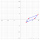What is this The area of a parallelogram that has vertices with the coordinates (0, 0), (4, 0),(5, 3), and (1, 3)?
• Trapezoid MO-5-Z8ABCD is a trapezoid that lime segment CE is divided into a triangle and parallelogram, as shown. Point F is the midpoint of CE, DF line passes through the center of the segment BE, and the area of the triangle CDE is 3 cm2. Determine the area of the trape
• There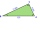There is a triangle ABC: A (-2,3), B (4, -1), C (2,5). Determine the general equations of the lines on which they lie: a) AB side, b) height to side c, c) Axis of the AB side, d) median ta to side a
• The coordinates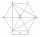The coordinates (5, 2) and (-6, 2) are vertices of a hexagon. Explain how to find the length of the segment formed by these endpoints. How long is the segment?
• Coordinates of a centroindLet’s A = [3, 2, 0], B = [1, -2, 4] and C = [1, 1, 1] be 3 points in space. Calculate the coordinates of the centroid of △ABC (the intersection of the medians).
• The triangleThe triangle is given by three vertices: A [0.0] B [-4.2] C [-6.0] Calculate V (intersection of heights), T (center of gravity), O - center of a circle circumscribed
• Line intersect segment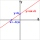Decide whether the line p : x + 2 y - 7 = 0 intersects the line segment given by points A[1, 1] and B[5, 3]
• CircleThe circle touches two parallel lines p and q, and its center lies on line a, which is the secant of lines p and q. Write the equation of the circle and determine the coordinates of the center and radius. p: x-10 = 0 q: -x-19 = 0 a: 9x-4y+5 = 0
• MO - trianglesOn the AB and AC sides of the triangle ABC lies successive points E and F, on segment EF lie point D. The EF and BC lines are parallel and is true this ratio FD:DE = AE:EB = 2:1. The area of ABC triangle is 27 hectares and line segments EF, AD, and DB seg
• Vertices of a right triangleShow that the points D(2,1), E(4,0), F(5,7) are vertices of a right triangle.
• Segment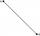Calculate the segment AB's length if the coordinates of the end vertices are A[10, -4] and B[5, 5].
• Construct 8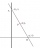Construct an analytical geometry problem where it is asked to find the vertices of a triangle ABC: the vertices of this triangle must be the points A (1,7) B (-5,1) C (5, -11). the said problem should be used the concepts of: distance from a point to a li
• Right triangle from axesA line segment has its ends on the coordinate axes and forms with them a triangle of area equal to 36 square units. The segment passes through the point ( 5,2). What is the slope of the line segment?